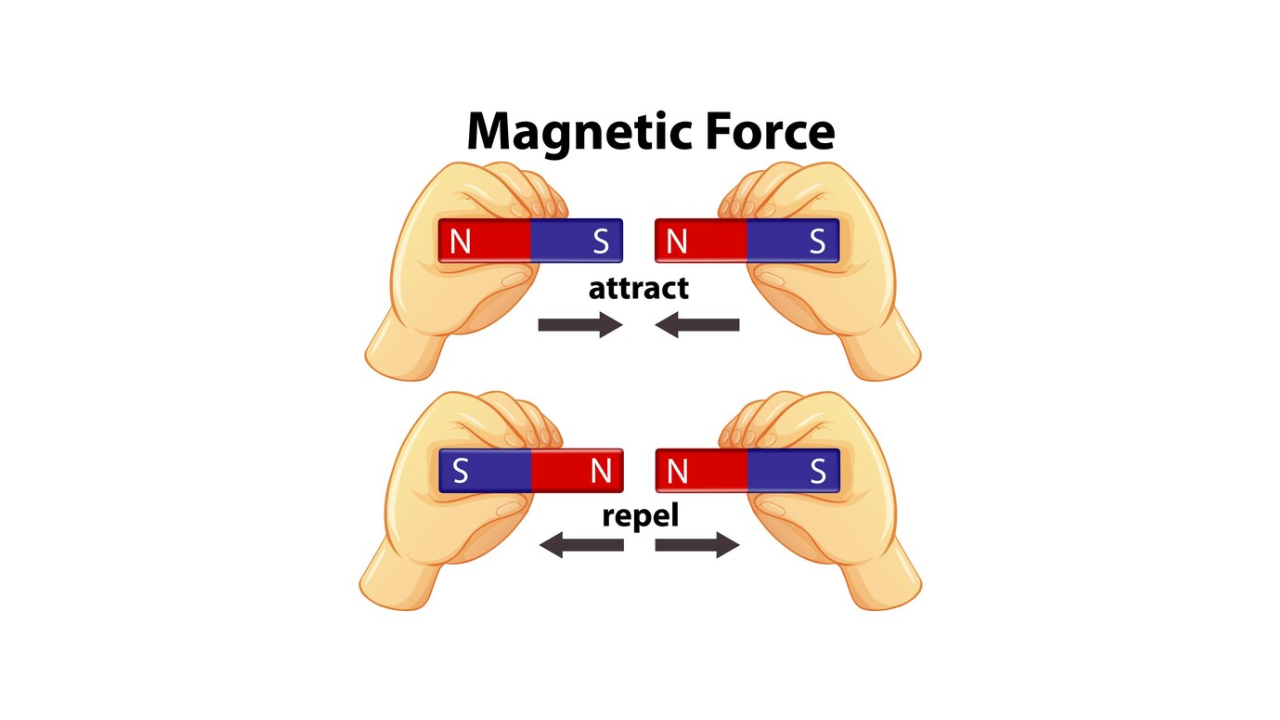What is Magnetic Flux?

The magnetic flux is the collection of force lines that extend from the north pole to the south pole of a magnet. It is represented by the symbol Φ (the lowercase Greek letter phi). The strength of a magnetic field is indicated by the number of magnetic flux lines, and factors influencing this strength include the material, physical geometry, and distance from the magnet. Magnetic field lines are more concentrated at the poles.The unit of magnetic flux is the Weber (Wb), where one Weber is equivalent to a certain number of lines. Due to the largeness of the weber, the microweber (µWb) is commonly used in practical situations, with one microweber equal to 100 lines of magnetic flux.

What is Magnetic Flux Density (B)

Magnetic flux density, symbolized as B, quantifies the amount of flux per unit area perpendicular to the magnetic field. The unit for magnetic flux density is the tesla (T), where one tesla equals one Weber per square meter. The formula expressing flux density is:

Where:

• is the magnetic flux density in teslas (T),
• is the flux in webers (Wb),
• is the cross-sectional area in square meters of the magnetic field.
Share.
error: Content is protected !!